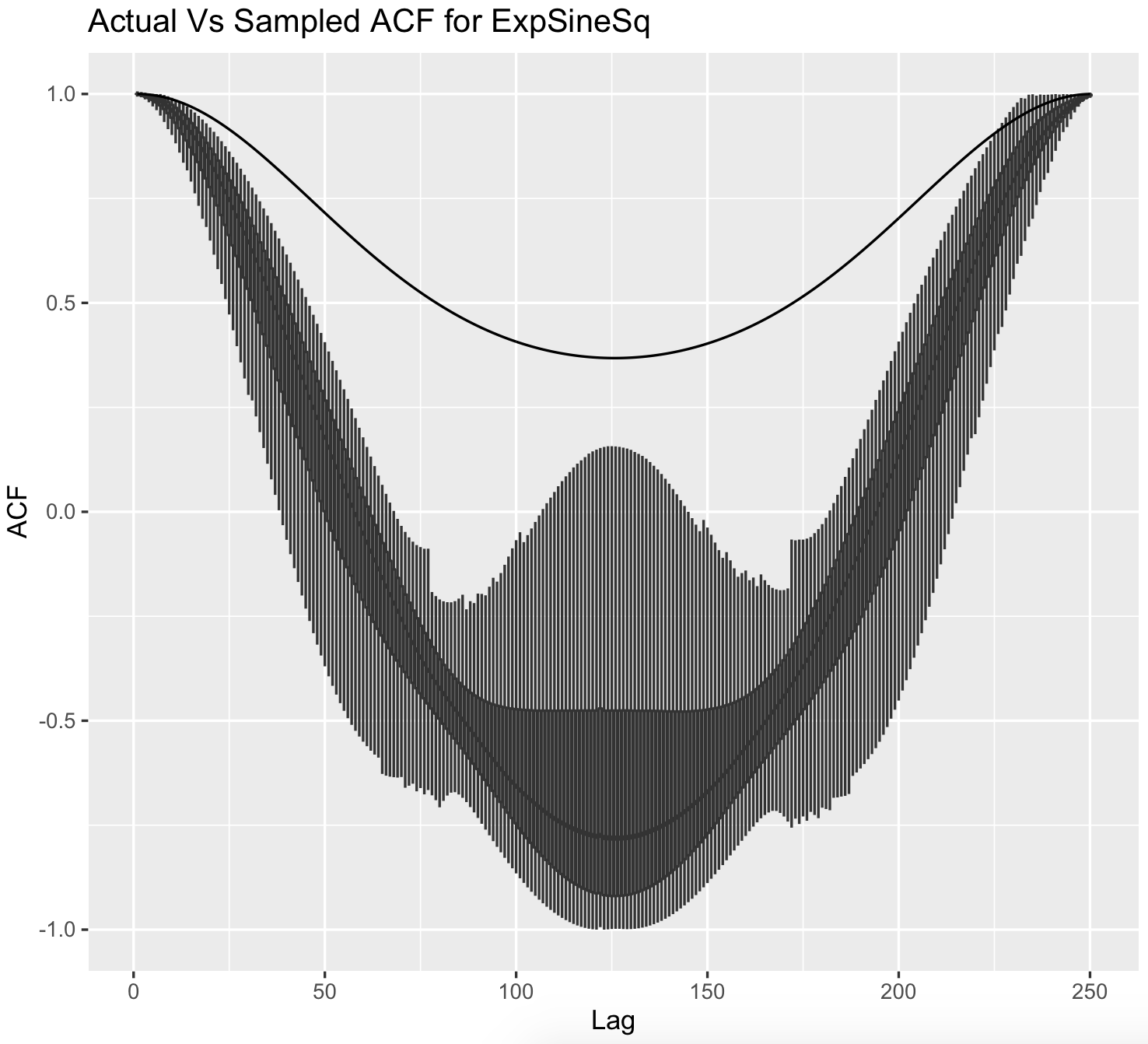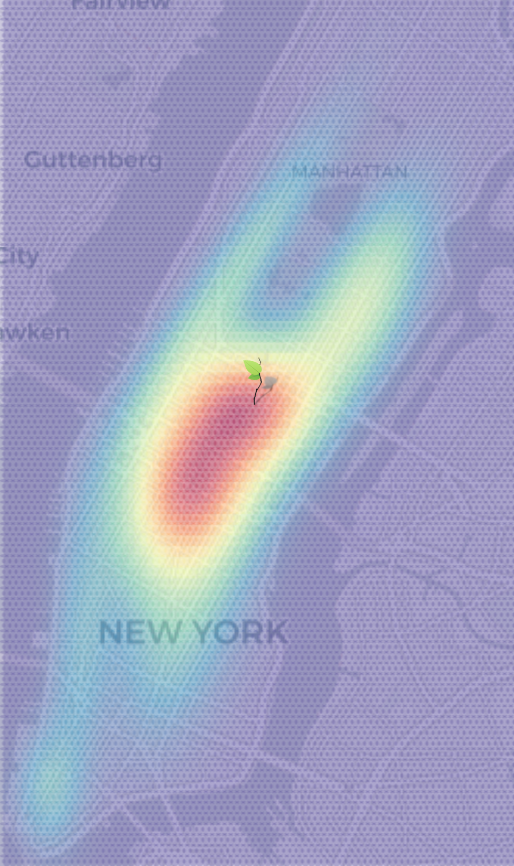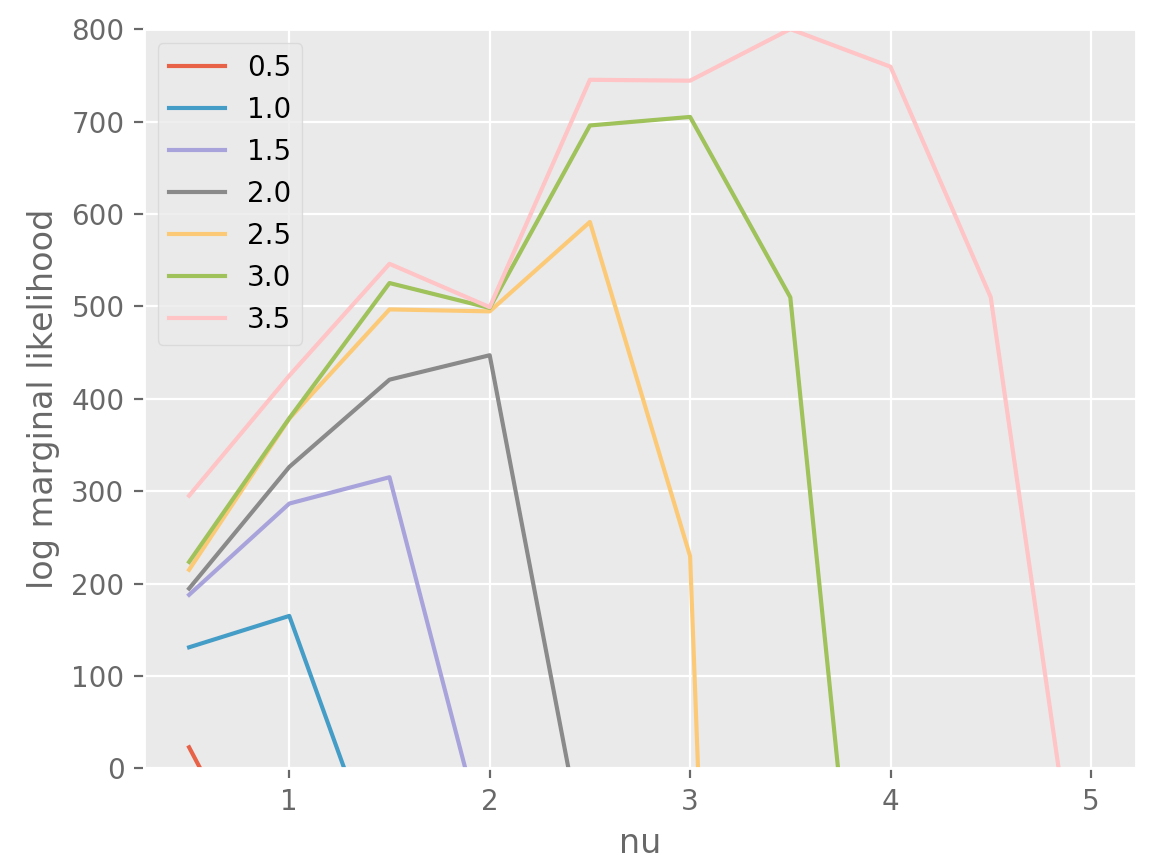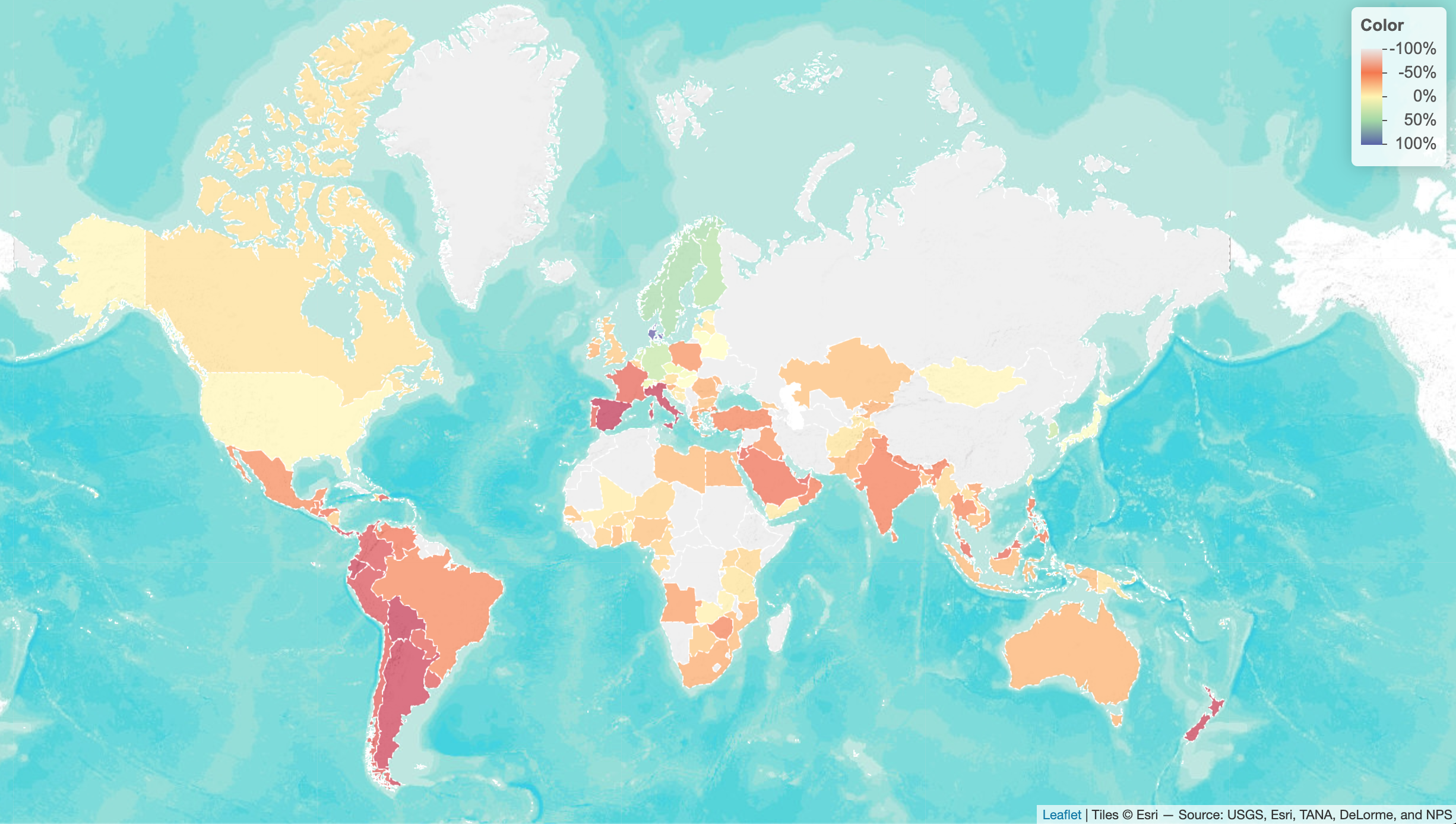# Random Projects

## Inferring Gaussian Process Autocorrelation

It seems reasonable and intuitive to think that the sample autocovariance function of a stationary gaussian process to be a sufficient statistic for its covariance function, and I read that this is indeed true for certain stationary GPs with a rational spectrum. This condition is quite similar (if not the same) for GPs to possess a state space representation.

It’s also interesting that not all GPs are ergodic. GPs are mixing (and hence ergodic, I believe) if the covariance dies off to zero after a point, or if its spectrum is absolutely continuous. Loosely, this means that the distribution of the process can be inferred from just one long sample. GPs with an exponentiated sine squared (ESS) covariance function, for example, wouldn’t be ergodic.

As a consequence, the covariance function of a zero mean GP with an ESS kernel, and similar signals, cannot be inferred from a single sample. This is reasonable, as no matter how long the signal is, there’s no new information in it after a certain point. Intuitively, a sample from a zero mean GP with an ESS kernel might look like $$(3, 2.5, 3, 2.5, ...)$$. The ESS is a kernel which is periodic, and the correlation of points spaced half a period apart is closest to zero (compared to any other pair of points), but still strictly positive. Another sample from that GP may look like $$(-2, -1.7, -2, -1.7, ...)$$.

Points one and two are closer together within each sample than across samples due to the correlation, but given just one observation of the signal (and with no knowledge of the mean of the process), it would appear that points one and two are negatively correlated.

The image below shows this; the black line is the true autocovariance function of the zero mean GP with an ESS kernel, and the boxplots show the sampling distribution of the unbiased sample autocovariance function based on single samples.## New York Conditional Taxi Dropoff Probabilities

I fit a twenty component mixture of multivariate normals, using scikit-learn, to the four dimensional new york taxi pickup/dropoffs dataset.

The dimensions look like (pickup_lat, pickup_lon, dropoff_lat, dropoff_lon). The aim is to predict the distribution of (dropoff_lat, dropoff_lon) by conditioning on (pickup_lat, pickup_lon).

Fancy ways to do this might include fitting a neural net or some kind of a gp to the conditional density, but here, I literally just fit a 4d mvn mixture to the whole dataset. To condition, we just plug in the pickup position and renormalize (Bayes rule).## Envelope Modelling

Google’s Quick Draw dataset contains multiple observations of quickly drawn envelopes. I fit a 256-component restricted boltzmann machine (heavily overparameterised; not a great model - I know) to the data, which represents a big nasty distribution over the random field that represents an envelope image. Now, starting off with a completely random image, using Gibbs sampling, we can make our way to the typical set of the distribution, which hopefully looks like an envelope. Here’s what the burn in looks like:## Inferring the Extent of Differentiability

Let’s say that we have an observation of a noiseless function but we don’t know how smooth it is. You could probably fit a Matern GP with different smoothness parameters to see which parameter maximises the log marginal likelihood (the matern parameter corresponds to the number of times one can differentiate a sample from the gp).

Below, I’ve simulated a Matern GP with a particular parameter, and fit it using parameters ranging from $$\\{0.5, ..., 5\\}$$. The color & label correspond to the parameter while sampling.## Modelling Audio using GPs

I used the S-PAD and the GP-PAD models from Richard Turner’s thesis to make these plots using some random audio data from the internet.

Sample stan code for this.## Modelling my d20 dice

I fit a spline on a sphere representing my d20. The color represents the model probabilities. The distance from the centre represents the proportion of times my dice fell on a particular number during six hundred trials.Code to help with this (messy).

## Changes in Park-Going

… w.r.t. baseline, as a result of the pandemic (as of 23rd Apr 2020). Based on the Google mobility dataset.## Minimal Working Implementation of the Griffin Lim Algorithm

This code is based on the Librosa source on GitHub.

GLA

## 2020

### Astrophotography

I used to do a fair bit of astrophotography in university - it’s harder to find good skies now living in the city. Here are some of my old pictures. I’ve kept making rookie mistakes (too much ISO, not much exposure time, using a slow lens, bad stacking, …), for that I apologize!

### Probabilistic PCA

I’ve been reading about PPCA, and this post summarizes my understanding of it. I took a lot of this from Pattern Recognition and Machine Learning by Bishop.

### Modelling with Spotify Data

The main objective of this post was just to write about my typical workflow and views rather than come up with a great model. The structure of this data is also outside my immediate domain so I thought it’d be fun to write up a small diary on making a model with it.

# Random Projects

### Morphing with GPs

The main aim here was to morph space inside a square but such that the transformation preserves some kind of ordering of the points. I wanted to use it to generate some random graphs on a flat surface and introduce spatial deformation to make the graphs more interesting.

### SEIR Models

I had a go at a few SEIR models, this is a rough diary of the process.

### Speech Synthesis

The initial aim here was to model speech samples as realizations of a Gaussian process with some appropriate covariance function, by conditioning on the spectrogram. I fit a spectral mixture kernel to segments of audio data and concatenated the segments to obtain the full waveform. Partway into writing efficient sampling code (generating waveforms using the Gaussian process state space representation), I realized that it’s actually quite easy to obtain waveforms if you’ve already got a spectrogram.

### Efficient Gaussian Process Computation

I’ll try to give examples of efficient gaussian process computation here, like the vec trick (Kronecker product trick), efficient toeliptz and circulant matrix computations, RTS smoothing and Kalman filtering using state space representations, and so on.

## 2019

### Gaussian Process Middle C

First of my experiments on audio modeling using Gaussian processes. Here, I construct a GP that, when sampled, plays middle c the way a grand piano would.

Consider: